Area of combination of figures : circle based

Chapter 12 Class 10 Areas related to Circles
Concept wise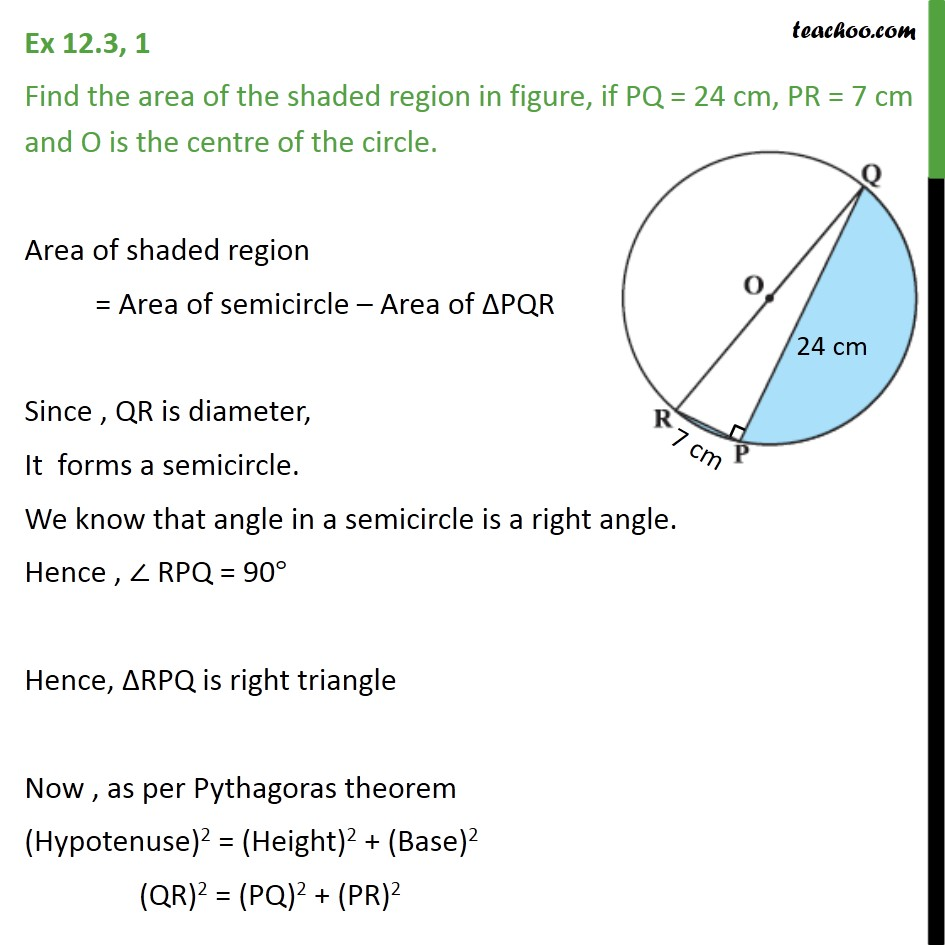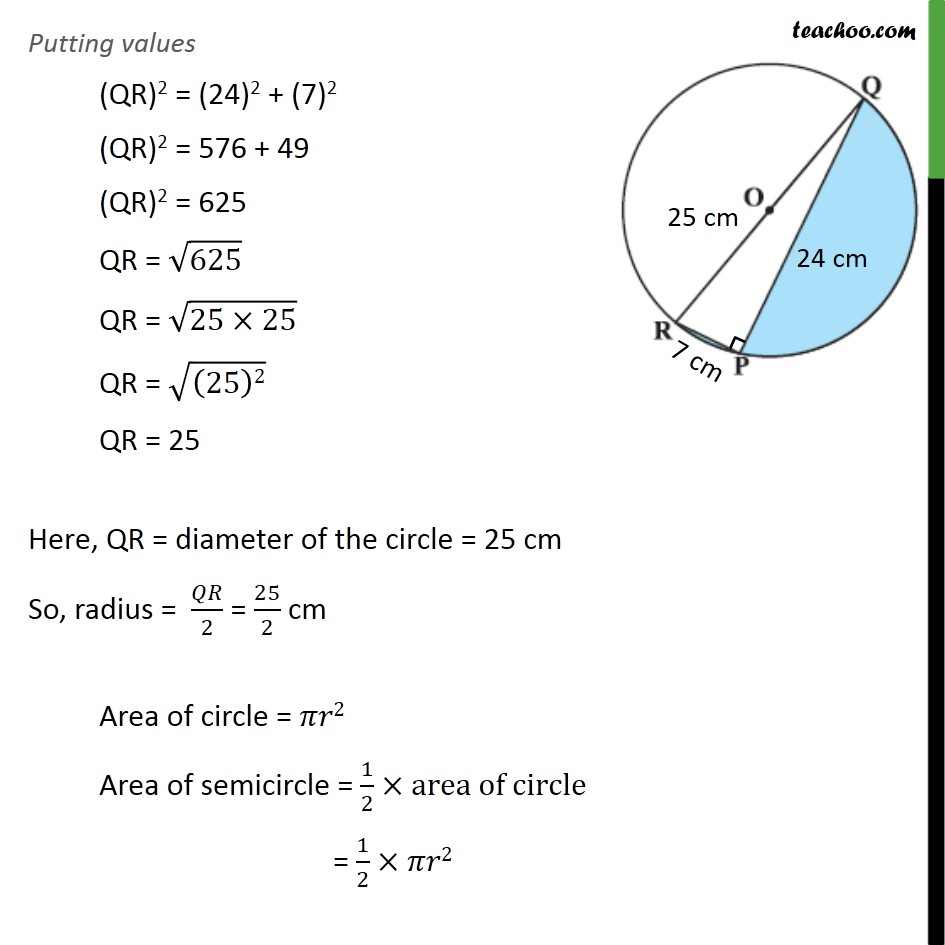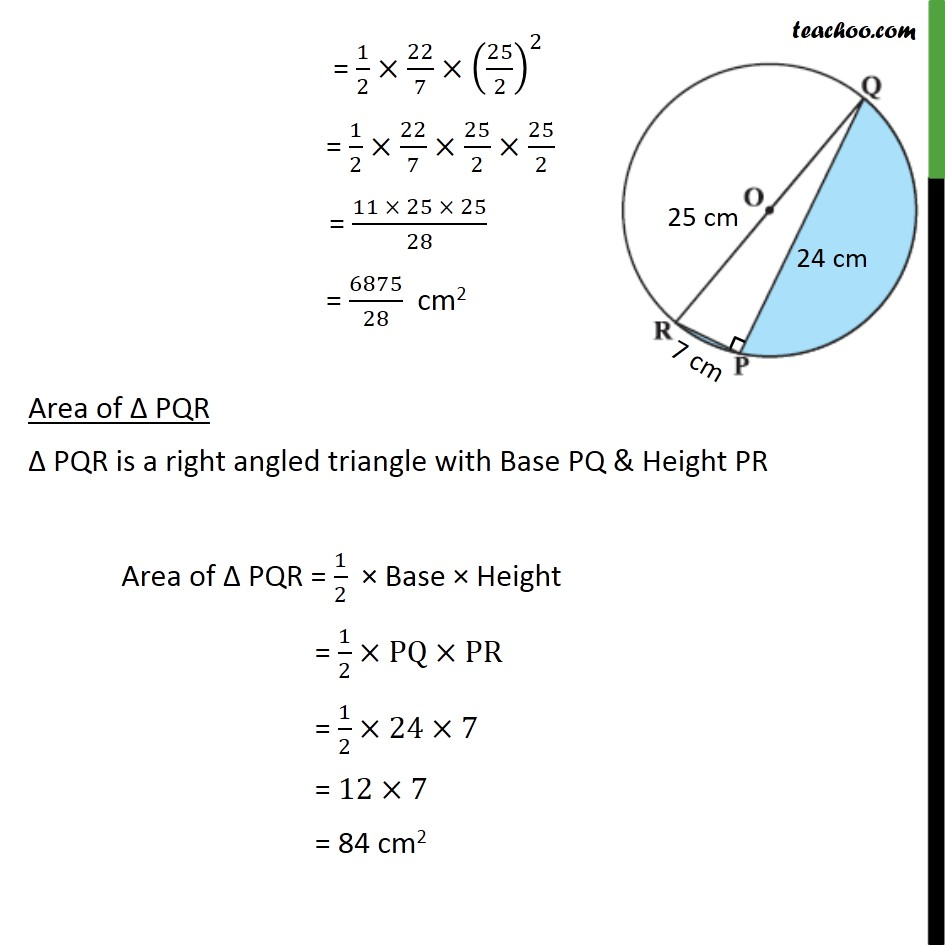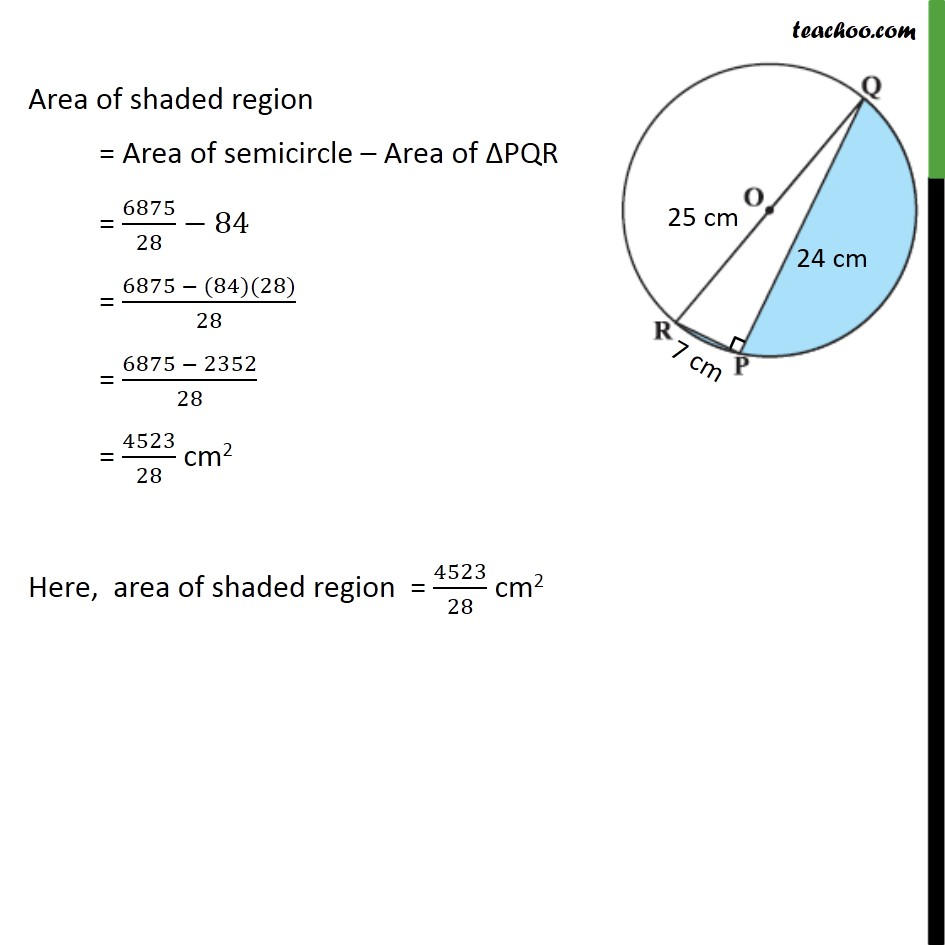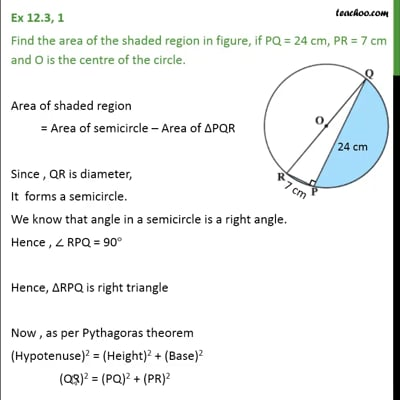This video is only available for Teachoo black users

Solve all your doubts with Teachoo Black (new monthly pack available now!)

### Transcript

Ex 12.3, 1 Find the area of the shaded region in figure, if PQ = 24 cm, PR = 7 cm and O is the centre of the circle. Area of shaded region = Area of semicircle – Area of ΔPQR Since , QR is diameter, It forms a semicircle. We know that angle in a semicircle is a right angle. Hence , ∠ RPQ = 90° Hence, ΔRPQ is right triangle Now , as per Pythagoras theorem (Hypotenuse)2 = (Height)2 + (Base)2 (QR)2 = (PQ)2 + (PR)2 Putting values (QR)2 = (24)2 + (7)2 (QR)2 = 576 + 49 (QR)2 = 625 QR = √625 QR = √(25×25) QR = √((25)2) QR = 25 Here, QR = diameter of the circle = 25 cm So, radius = 𝑄𝑅/2 = 25/2 cm Area of circle = 𝜋𝑟2 Area of semicircle = 1/2×area of circle = 1/2×𝜋𝑟2 = 1/2×22/7×(25/2)^2 = 1/2×22/7×25/2×25/2 = (11 × 25 × 25)/28 = 6875/28 cm2 Area of Δ PQR Δ PQR is a right angled triangle with Base PQ & Height PR Area of Δ PQR = 1/2 × Base × Height = 1/2×PQ×PR = 1/2×24×7 = 12×7 = 84 cm2 Area of shaded region = Area of semicircle – Area of ΔPQR = 6875/28− 84 = (6875 − (84)(28))/28 = (6875 − 2352)/28 = 4523/28 cm2 Here, area of shaded region = 4523/28 cm2